# Skew polygon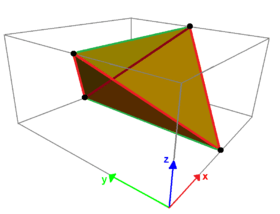The (red) side edges of tetragonal disphenoid represent a regular zig-zag skew quadrilateral.

In geometry, a skew polygon is a polygon whose vertices are not all coplanar. Skew polygons must have at least 4 vertices. The interior surface (or area) of such a polygon is not uniquely defined.

Skew infinite polygons (apeirogons) have vertices which are not all collinear.

A zig-zag skew polygon or antiprismatic polygon has vertices which alternate on two parallel planes, and thus must be even-sided.

Regular skew polygon in 3 dimensions (and regular skew apeirogons in 2 dimensions) are always zig-zag.

## Antiprismatic skew polygon in 3 dimensions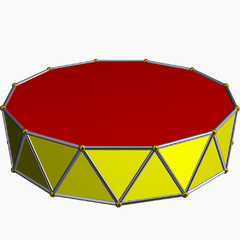A uniform n-gonal antiprism has a 2n-sided regular skew polygon defined along its side edges.

A regular skew polygon is isogonal with equal edge lengths. In 3 dimensions a regular skew polygon is a zig-zag skew (or antiprismatic polygon), with vertices alternating between two parallel planes. The sides of an n-antiprism can define a regular skew 2n-gons.

A regular skew n-gonal can be given a symbol {p}#{ } as a blend of a regular polygon, {p} and an orthogonal line segment, { }. The symmetry operation between sequential vertices is glide reflection.

Examples are shown on the uniform square and pentagon antiprisms. The star antiprisms also generate regular skew polygons with different connection order of the top and bottom polygons.

 Skew square Skew hexagon Skew octagon Skew decagon Skew dodecagon {2}#{ } {3}#{ } {4}#{ } {5}#{ } {5/2}#{ } {5/3}#{ } {6}#{ }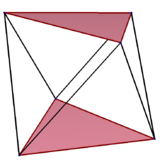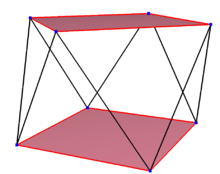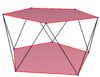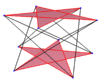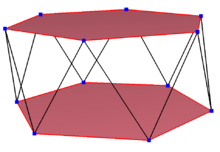s{2,4} s{2,6} s{2,8} s{2,10} sr{2,5/2} s{2,10/3} s{2,12}

A regular compound skew 2n-gon can be similarly constructed by adding a second skew polygon by a rotation. These shares the same vertices as the prismatic compound of antiprisms.

 Skew squares Skew hexagons Skew decagons Two {2}#{ } Three {3}#{ } Two {3}#{ } Two {5/3}#{ }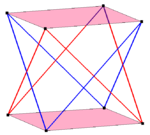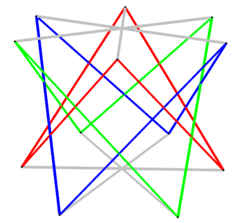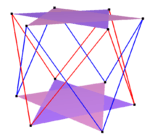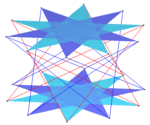Petrie polygons are regular skew polygons defined within regular polyhedra and polytopes. For example, the 5 Platonic solids have 4, 6, and 10-sided regular skew polygons, as seen in these orthogonal projections with red edges around the projective envelope. The tetrahedron and octahedron include all the vertices in the zig-zag skew polygon and can be seen as a digonal and a triangular antiprisms respectively.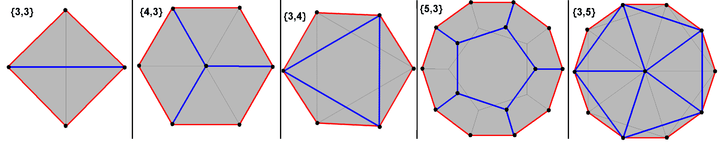The regular skew polyhedron have regular faces, and regular skew polygon vertex figures. Three are infinite space-filling in 3-space and others exist in 4-space, some within the uniform 4-polytope.

Skew vertex figures of the 3 infinite regular skew polyhedra
{4,6|4} {6,4|4} {6,6|3}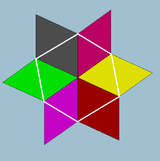Regular skew hexagon
{3}#{ }Regular skew square
{2}#{ }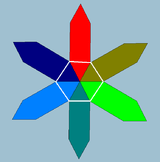Regular skew hexagon
{3}#{ }

### Isogonal skew polygons in 3 dimensions

An isogonal skew polygon is a skew polygon with one type of vertex, connected by two types of edges. Isogonal skew polygons with equal edge lengths can also be considered quasiregular. It is similar to a zig-zag skew polygon, existing on two planes, except allowing one edge to cross to the opposite plane, and the other edge to stay on the same plane.

Isogonal skew polygons can be defined on even-sided n-gonal prisms, alternatingly following an edge of one side polygon, and moving between polygons. For example, on the vertices of a cube. Vertices alternate between top and bottom squares with red edges between sides, and blue edges along each side.

Octagon Dodecagon 24-gon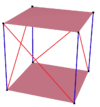Cube, square-diagonal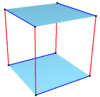Cube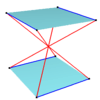Crossed cube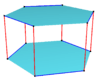Hexagonal prism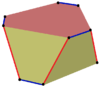Hexagonal prism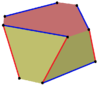Hexagonal prism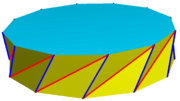Twisted prism

## Regular skew polygons in 4 dimensions

In 4 dimensions a regular skew polygon can have vertices on a Clifford torus and related by a Clifford displacement. Unlike zig-zag skew polygons, skew polygons on double rotations can include an odd-number of sides.

The petrie polygons of the regular 4-polytope define regular skew polygons. The Coxeter number for each coxeter group symmetry expresses how many sides a petrie polygon has. This is 5 sides for a 5-cell, 8 sides for a tesseract and 16-cell, 12 sides for a 24-cell, and 30 sides for a 120-cell and 600-cell.

When orthogonally projected onto the Coxeter plane these regular skew polygons appear as regular polygon envelopes in the plane.

A4, [3,3,3] B4, [4,3,3] F4, [3,4,3] H4, [5,3,3]
Pentagon Octagon Dodecagon Triacontagon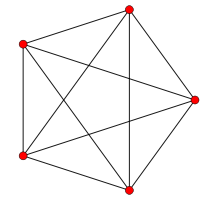5-cell
{3,3,3}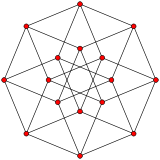tesseract
{4,3,3}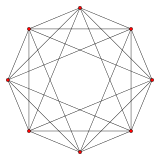16-cell
{3,3,4}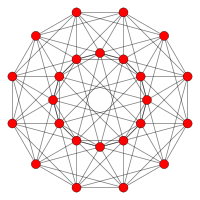24-cell
{3,4,3}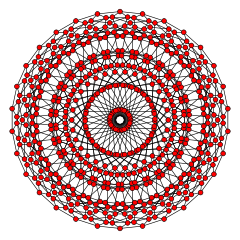120-cell
{5,3,3}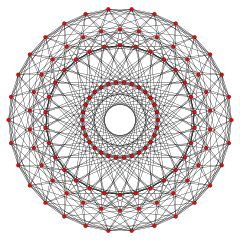600-cell
{3,3,5}

The n-n duoprism and dual duopyramids also have 2n-gonal petrie polygons. (The tesseract is a 4-4 duoprism, and the 16-cell is a 4-4 duopyramid.)

Hexagon Decagon Dodecagon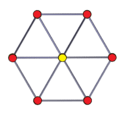3-3 duoprism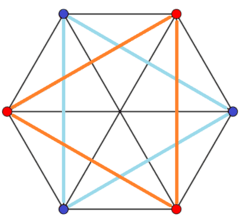3-3 duopyramid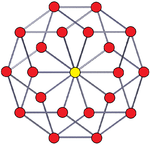5-5 duoprism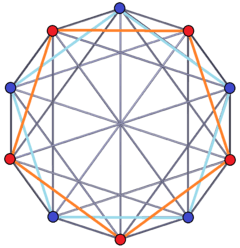5-5 duopyramid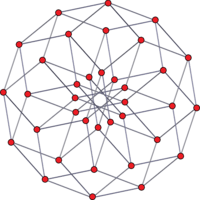6-6 duoprism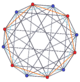6-6 duopyramid

## References

1. Regular complex polytopes , p. 6
2. Abstract Regular Polytopes, p.217
• McMullen, Peter; Schulte, Egon (December 2002), Abstract Regular Polytopes (1st ed.), Cambridge University Press, ISBN 0-521-81496-0 p. 25
• Williams, Robert (1979). The Geometrical Foundation of Natural Structure: A Source Book of Design. Dover Publications, Inc. ISBN 0-486-23729-X. "Skew Polygons (Saddle Polygons)." §2.2
• Coxeter, H.S.M.; Regular complex polytopes (1974). Chapter 1. Regular polygons, 1.5. Regular polygons in n dimensions, 1.7. Zigzag and antiprismatic polygons, 1.8. Helical polygons. 4.3. Flags and Orthoschemes, 11.3. Petrie polygons
• Coxeter, H. S. M. Petrie Polygons. Regular Polytopes, 3rd ed. New York: Dover, 1973. (sec 2.6 Petrie Polygons pp. 2425, and Chapter 12, pp. 213–235, The generalized Petrie polygon )
• Coxeter, H. S. M. & Moser, W. O. J. (1980). Generators and Relations for Discrete Groups. New York: Springer-Verlag. ISBN 0-387-09212-9. (1st ed, 1957) 5.2 The Petrie polygon {p,q}.
• John Milnor: On the total curvature of knots, Ann. Math. 52 (1950) 248–257.
• J.M. Sullivan: Curves of finite total curvature, ArXiv:math.0606007v2